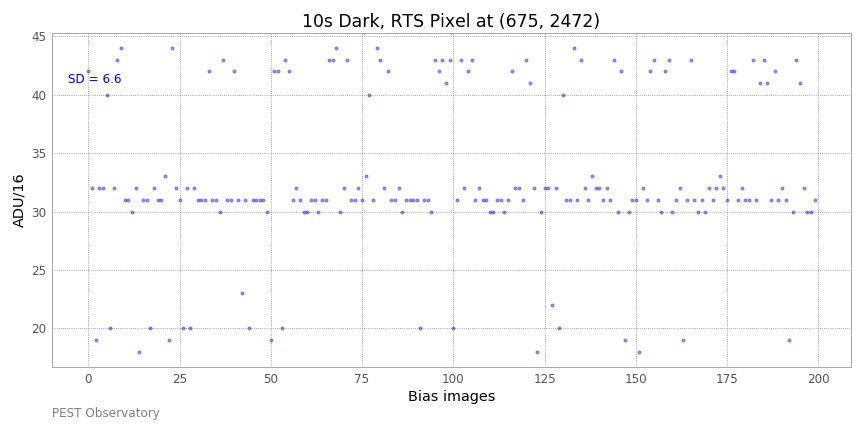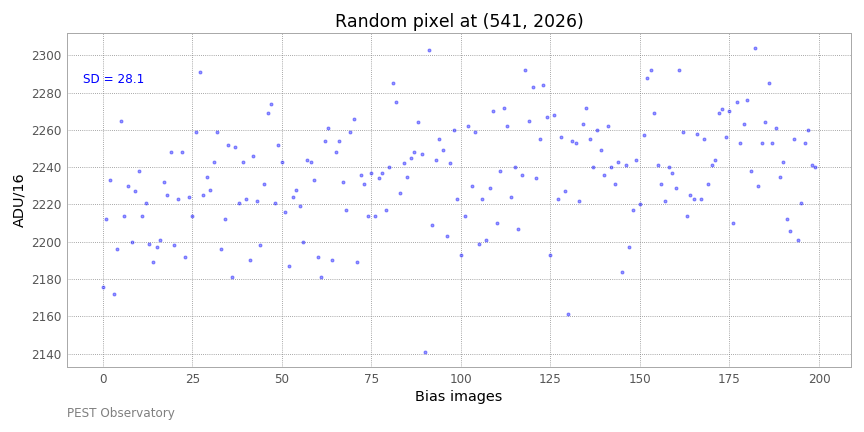# RTS Pixel Behaviour

### Articles in this series:

Characterising RTS

Dark Frames

Linearity

Binning

On-sky Photometry

We saw in the previous page that there are some pixels on the CMOS sensor which, on bias frames, tend to jump between 3 distinct levels e.g. 10 ADUs above or below the normal bias level.

I want to see how these same pixels respond with longer dark exposures, and when the sensor is exposed to light.

### RTS pixel in dark frame

I acquired 200 dark frames with 10s exposure, then plotted the ADUs of a number of those RTS pixels identified before.  Here’s how the pixel at (675,  2472) behaved with 10s dark exposure.This pixel pretty much behaves the same when there is no light, whether the exposure is 10s, or 0.001s as in the bias frames.  I checked that this is true of the other RTS pixels identified as well.

### RTS Pixel in Light Frame

I then acquired 200 light frames, also 10s exposures but with a light level enough to give about half full well, or about 2000 ADU.  Below is the same pixel under light;Notice that, if the RTS behaviour is still there it is not appreciable, at least not by eye.  This test was done in a room with artificial lighting, at night, and with sheets of paper placed over the camera nosepiece to get the desired ADU level.  There was about an increase of about 20 ADU over the image acquisition period, so despite my attempt to keep the light level constant it looks like there was about a 1% increase in brightness.

Perhaps the RTS behaviour results in RTS pixels having higher scatter than others?  Below is a plot of a randomly chosen pixel;The slightly increasing trend is apparent here as well.  And interestingly the scatter (std deviation = 28.1) is actually worse than the RTS pixel.  In fact the overall scatter over each light frame is ~29 ADU.  This number is nudged higher by there being a number of hot pixels which should be corrected when dark subtraction is done.  So the RTS pixels apparently don’t have appreciably higher scatter than normal.

If my hypothesis that this is RTS, with stray electrons during the read process causing the jumps in level is true, and the phenomenon is still present even in the light frames, how much excess scatter would we expect to see?

### Calculation of excess scatter due to RTS

Assume Gain = 3.7 e-/ADU.  Then the 6.6 ADU scatter from RTS noise (top plot above) = 24.4 e-.

The number of electrons in each light frame pixel, corresponding to an ADU of 2240 = (2240-30)*3.7 = 8177 e-.  The expected SD from photon shot noise (Poisson noise, because light comes in discrete quanta) = SQRT(8177) = 90 e-, which is equivalent to 24 ADU.

The other source of noise is Read Noise.  From QHYCCD, this = 2.7 e-.

Therefore:

Without RTS, the expected scatter = SQRT(90^2 + 2.7^2) = 90.5 e- = 24.5 ADU.
With RTS, expected scatter = SQRT(90^2 + 2.7^2 + 24.4^2) = 93.7 e- = 25.3 ADU.

### Some conclusions

The calculation above was for a pixel affected by RTS.  But less than 1 in 1000 pixels are.  With the small 2.4 micron pixels, the pixel scale for my scope is just 0.3 arcsec/pix (12″ f/5).  I normally use a photometry aperture of radius ~7″.  So there will be about 1,700 pixels within this aperture and on average only 1 or 2 RTS affected pixels, adding 1-4 ADU total intensity.  I will be aiming for images with >1m total ADU intensity (sum of ADU for all the pixels within the aperture), so that the impact on photometry will be of the order of 1 ppm.  This is insignificant for ground based photometry.  Other sources of noise will swamp out RTS.

### Next tests

I will be looking at dark frames from the QHY183M next.  Do the hot pixels subtract out?  How about amp-glow?